Exchange Potential: LDA and GGA versus Exact Potential

## Exchange Potential: LDA and GGA versus Exact Potential

### I) Brief introduction

The exact multiplicative exchange potential of density functional theory can be obtained numerically via the optimized potential method (OPM) . The OPM results can be used to analyze the properties of approximate density functionals for the exchange energy, as the local density approximation (LDA) and the generalized gradient approximation (GGA). Below these approximations are compared with the exact potentials for a number of systems, ranging from atoms to solids. Sometimes also the Krieger-Li-Iafrate (KLI) approximation  to the exact exchange potential is displayed.

The units of the plots are as follows: All length scales are given in Bohr, the potentials are in Hartree.

### II) Results for atoms

#### II.1) Neon (GGA = PW91 )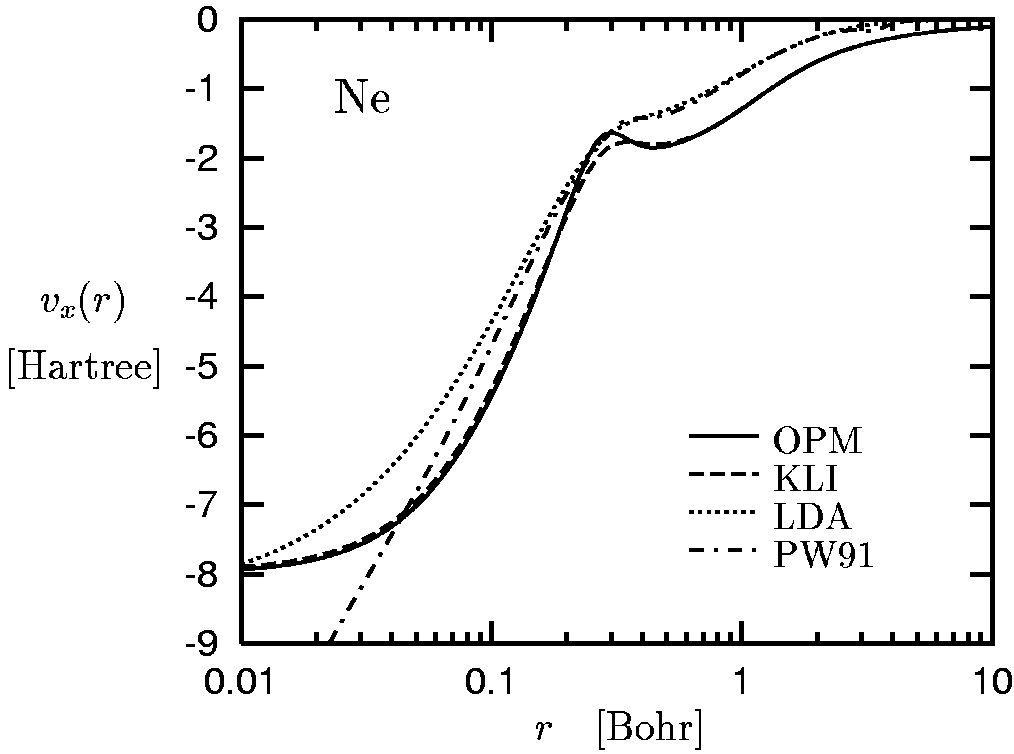• The atomic shell structure is obvious in the exact exchange potential (OPM).
• The exact exchange potential falls off like -1/r for large r, i.e. in the asymptotic region in which the density decays exponentially.
• The KLI approximation also shows the shell structure, although much less pronounced. It is exact in the asymptotic regime.
• Neither the LDA nor the GGA reproduce the shell structure. The LDA and GGA potentials decay exponentially for large r (with the exception of the B88-GGA potential  which formally decays as r-2 ).
• The GGA potential depends on the Laplacian of the density, which diverges like 1/r at the position of the nucleus (cusp condition).

see
E. Engel and S. H. Vosko, Phys. Rev. B 47, 13164 (1993).

#### II.3) Zinc

see E. Engel and R. M. Dreizler, J. Comput. Chem. 20, 31 (1999).

#### II.4) Spin-difference potentials for Manganese, Arsenic, Copper, Europium

see E. Engel and S. H. Vosko, Phys. Rev. A 47, 2800 (1993).

### III) Results for molecules

#### III.1) H2 (GGA = B88 )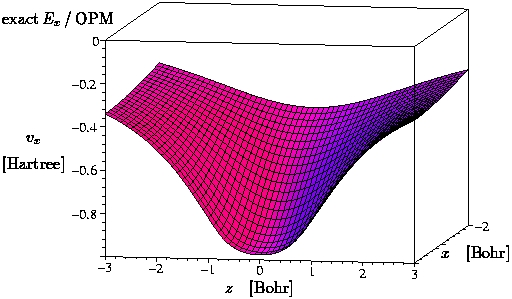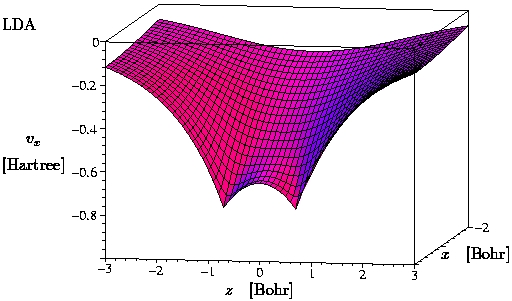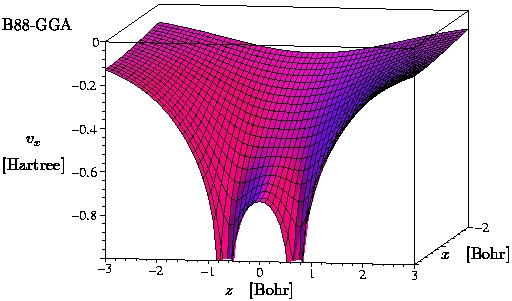• The plots show the potential in the x-z-plane. The atoms are sitting on the x-axis at z = +/-0.7 Bohr.
• The exact exchange potential of H2 only cancels the self-interaction component in the Hartree potential.

#### III.2) N2 (GGA = B88 )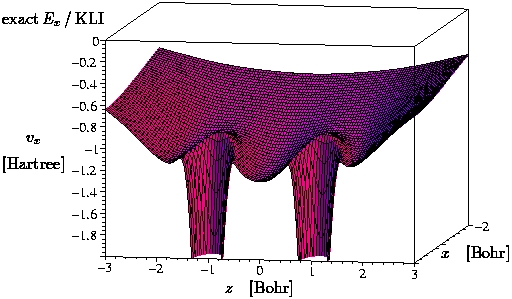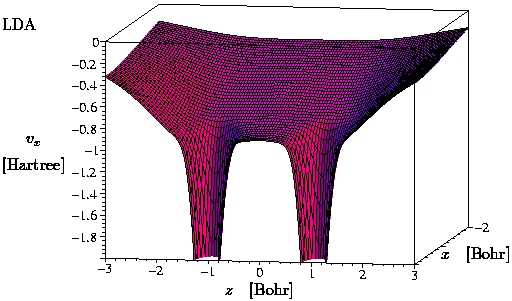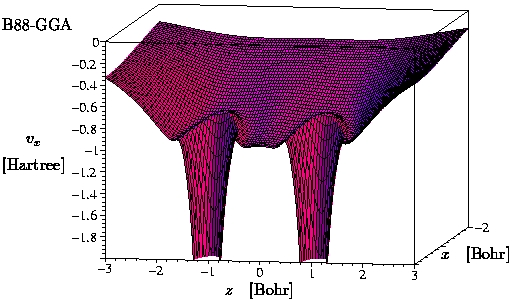### IV) Results for jellium spheres

see E. Engel and S. H. Vosko, Phys. Rev. B 50, 10498 (1994).

### V) Results for solids

#### V.1) Silicon

• GGA = PW91  and B88 
• Plane-wave pseudopotential calculation with a cut-off energy of 25 Rydberg, k-point sampling based on 19 special k-points (a = 10.2 Bohr).
• The plots show the potential along  direction of the diamond structure.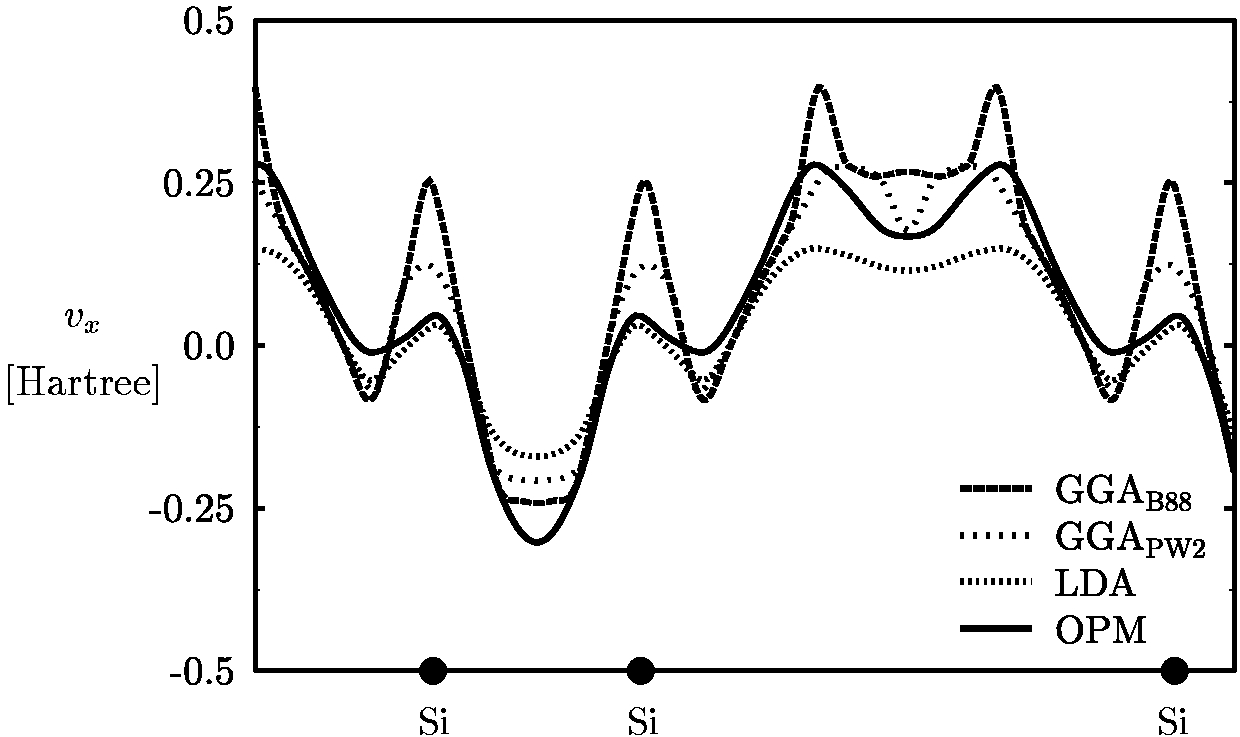#### V.2) Aluminum

• GGA = PW91 
• Plane-wave pseudopotential calculation with a cut-off energy of 100 Rydberg, k-point sampling based on 44 special k-points, 750 Kohn-Sham states included in evaluation of Kohn-Sham response function (a = 7.6 Bohr).
• The plots show the potential along  direction of the fcc structure.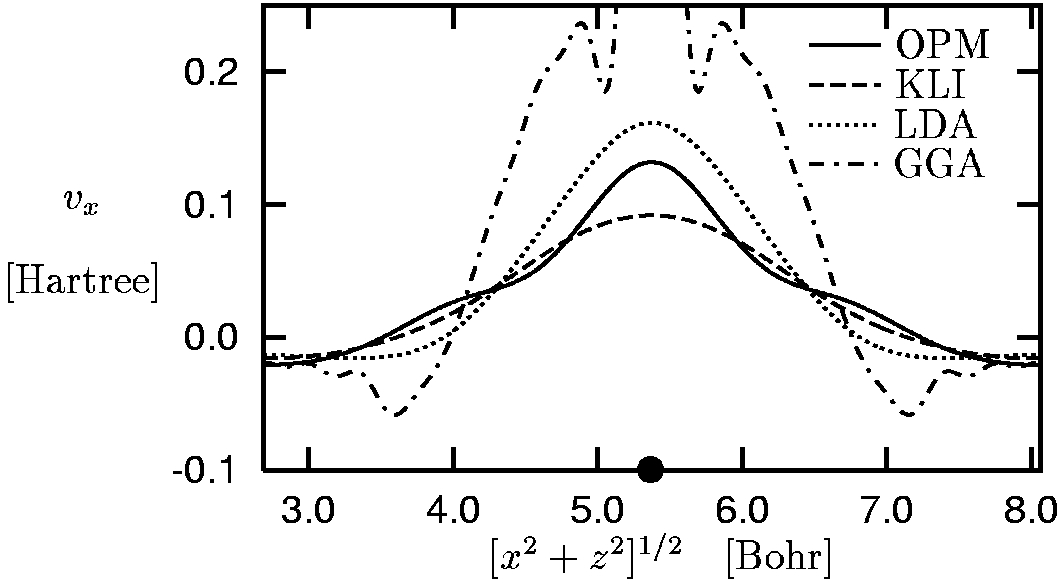• KLI approximation reasonably close to exact OPM result.
• GGA deviates much more from exact potential than LDA.

### References

1. J. D. Talman and W. F. Shadwick, Phys. Rev. A 14, 36 (1976).
2. J. B. Krieger, Y. Li, and G. J. Iafrate, Phys. Lett. A 146, 256 (1990).
3. J. P. Perdew, in Electronic Structure of Solids 1991, ed. by P. Ziesche and H. Eschrig (Akademie Verlag, Berlin, 1991), p.11.
4. A. D. Becke, Phys. Rev. A 38, 3098 (1988).
5. E. Engel, J. A. Chevary, L. D. Macdonald, and S. H. Vosko, Z. Phys. D 23, 7 (1992).

Home Top